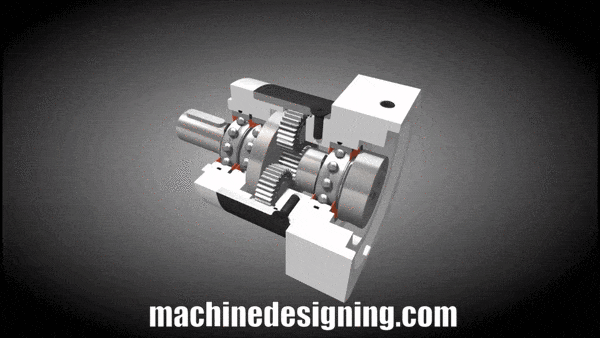# How To Size Planetary Reducer

In my previous posts, I said that I will explain the planetary reducer selection, later.As you know, planetary gearboxes have many types produced according to need.Helical gear planetary reducers can be used for precise positioning and low operating noise, and straight gear planetary reducers can be used for standard applications.Of course, these definition classifies reducers very simply. Because there are dozens of subspecies according to their use.For this article, let’s assume that we know the output torque, the speed of the system and the need for positioning precision.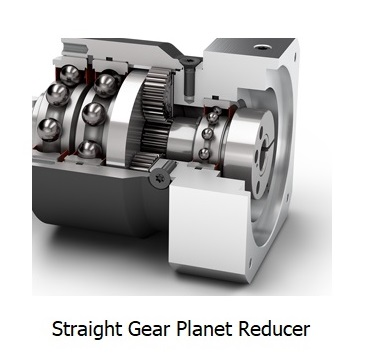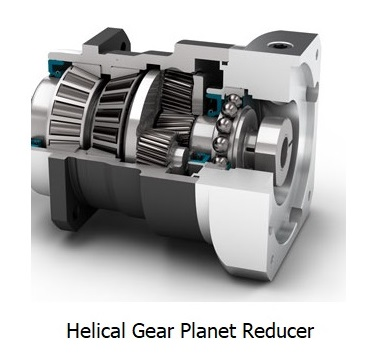We made the calculation for the system with Yaskawa SigmaJunmaSizer + and let’s assume that the system needs i:7 ratioand the maximum torque required by the system is 67Nm ( servo output 6,57Nm).

The reducer output should have a speed of 500 rpm and assume that the positioning accuracy is suitable up to 7 arc/min.I will also write about the calculation of positioning accuracy in my next posts.

#### Torque Check

First, let’s choose the size of the reducer that can give the required torque at this ratio.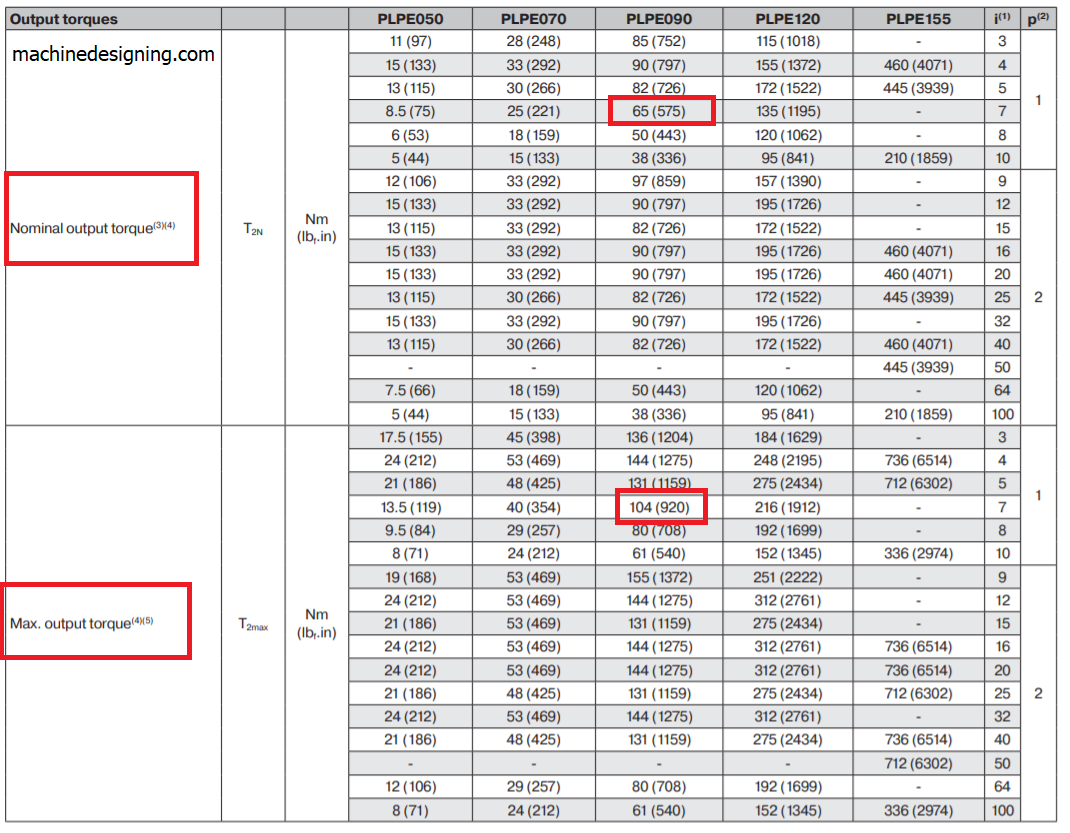As indicated in the image, the nominal output torque of the 90 size reducer is 65Nm and the maximum output torque is 104Nm.Since our system needs a maximum of 67Nm torque, we can conclude by comparing it with the maximum output torque value.The 90 size reducer is suitable for this job since the torque required by the system remains slightly above the nominal torque value.The average torque value will remain below the rated torque value during operation. This is a situation that we usually want when choosing planetary reducer.

#### Input Speed Check

The second value we need to check after determining that the torque value is suitable is the maximum input speed value.There are two different values to be aware of. The average thermal input speed (S1) if there is continuous operation, the maximum mechanical input speed (S6) if there is no continuous operation.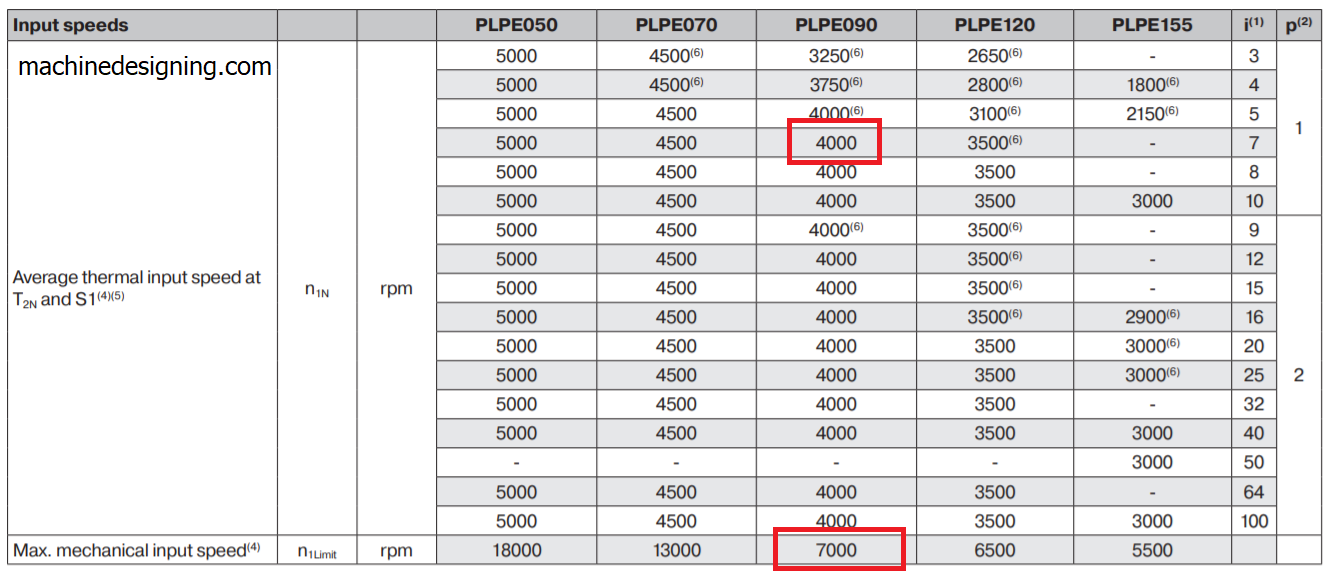Since 3500rpm input speed is sufficient for our system, there is no problem for our choose. ( for S1 4000rpm>3500rpm)

#### Axial and Radial Force Check

If load is to be connected directly to the output shaft of the reducer used in your application, you should check the axial and radial load values.When I work on machine designing , I generally prefer to use servo coupling between load and reducer.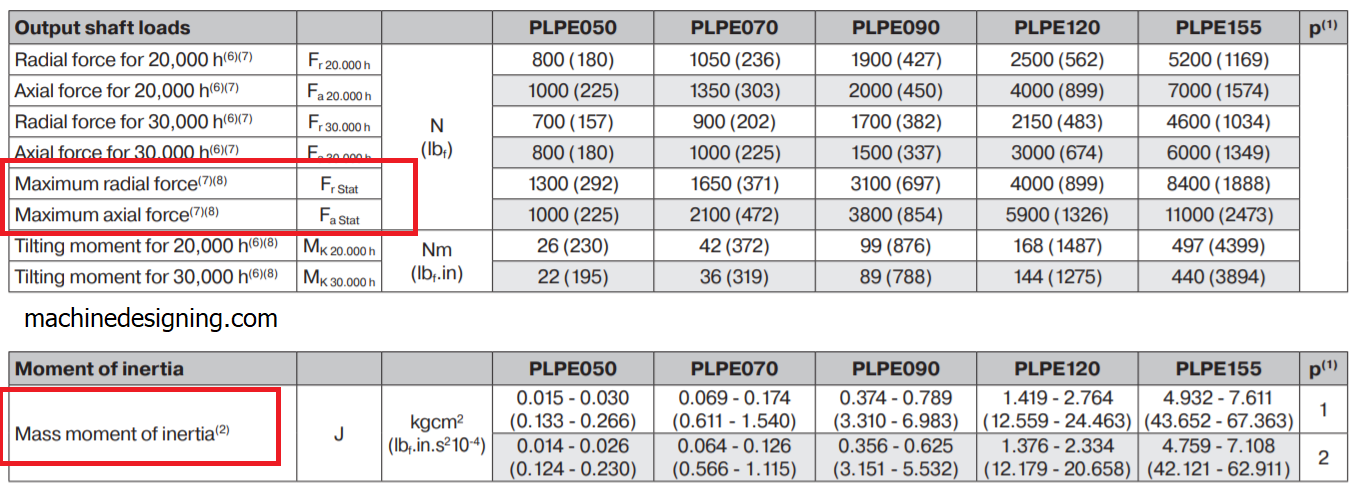#### Usage Mass Moment of Inertia of Reducer

In my previous posts, when choosing a servo motor with the Yaskawa program, I did not write exactly the inertia value of the reducer.However, I said that you should learn this value from the catalog while making a real calculation.As seen in the image above, there is a different internal inertia value for each body size.

#### Running Noise and Backlash Check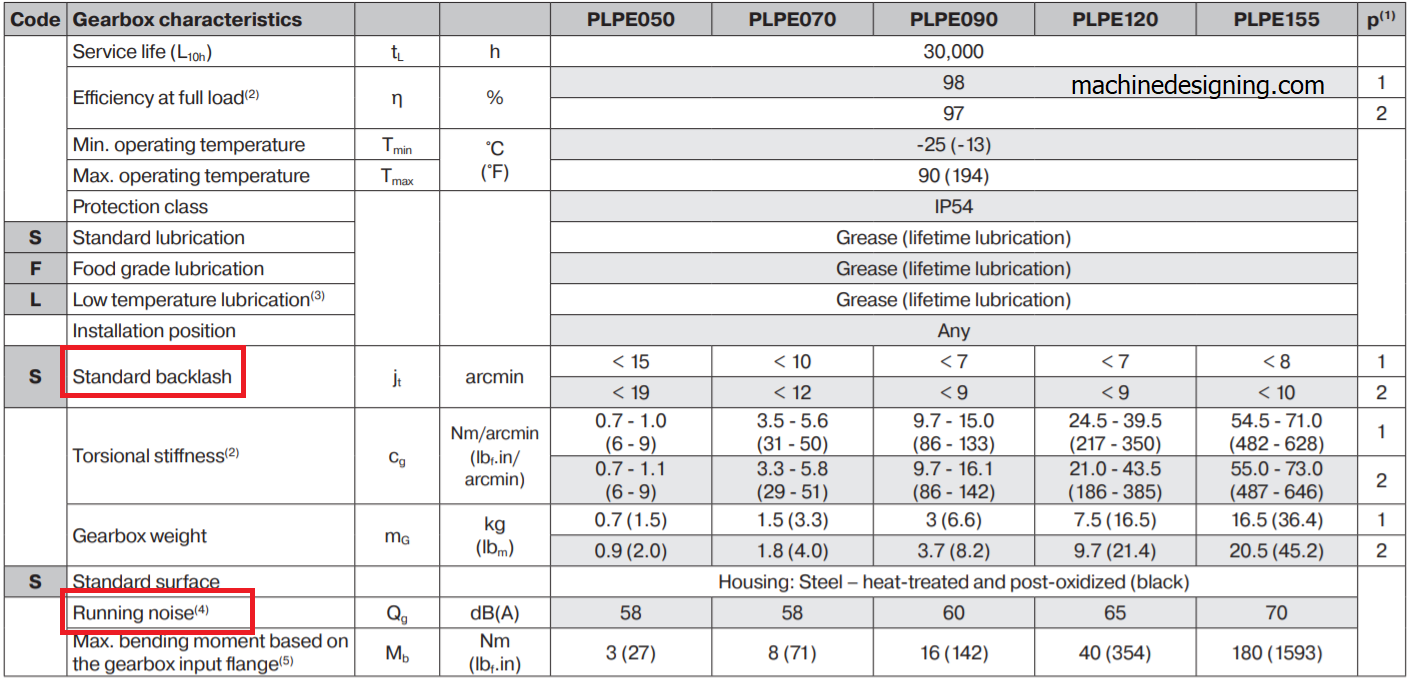Finally, after checking that the running noise and backlash values are suitable for your needs, you can place the 3D data of the reducer in your design.